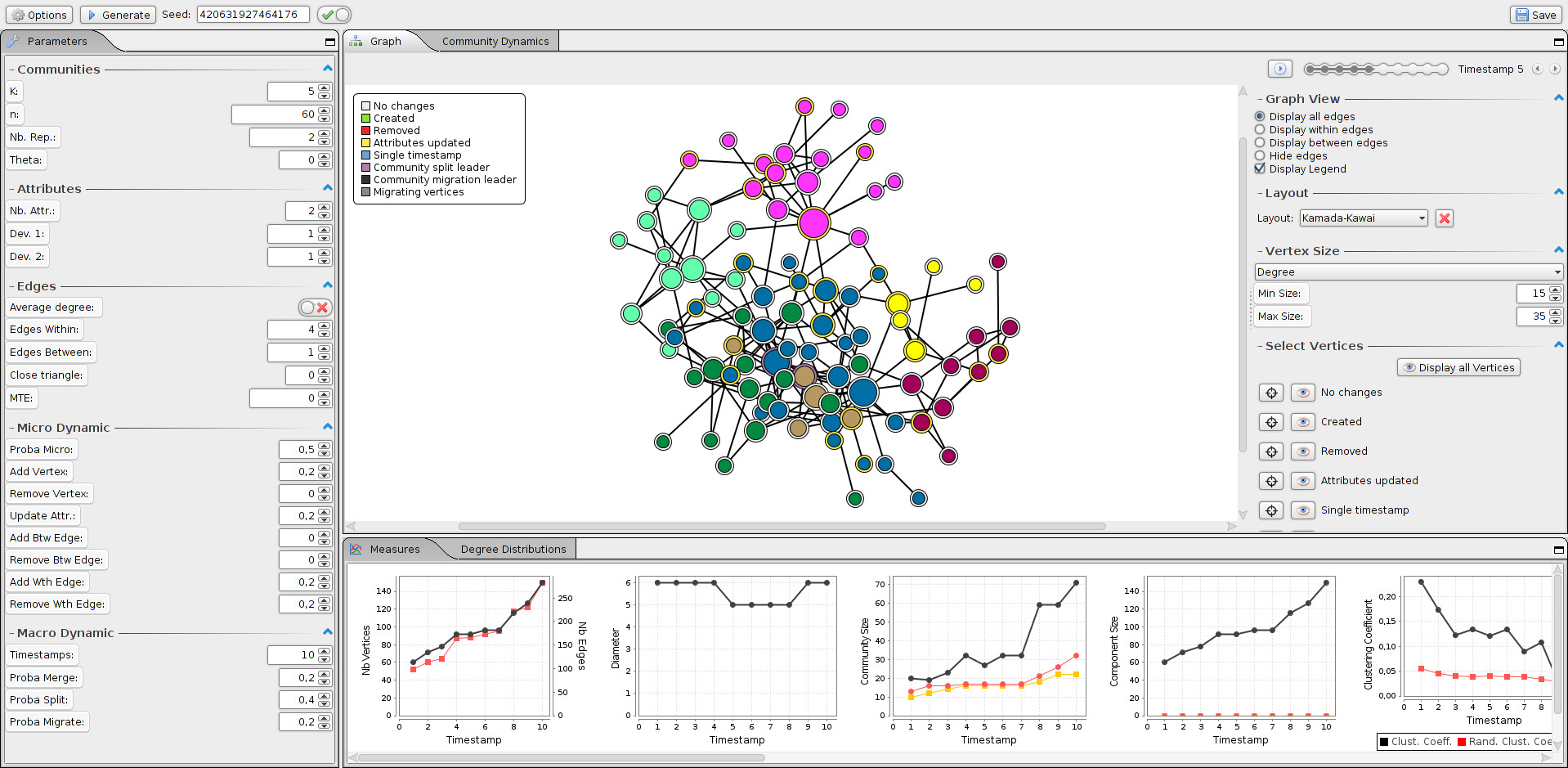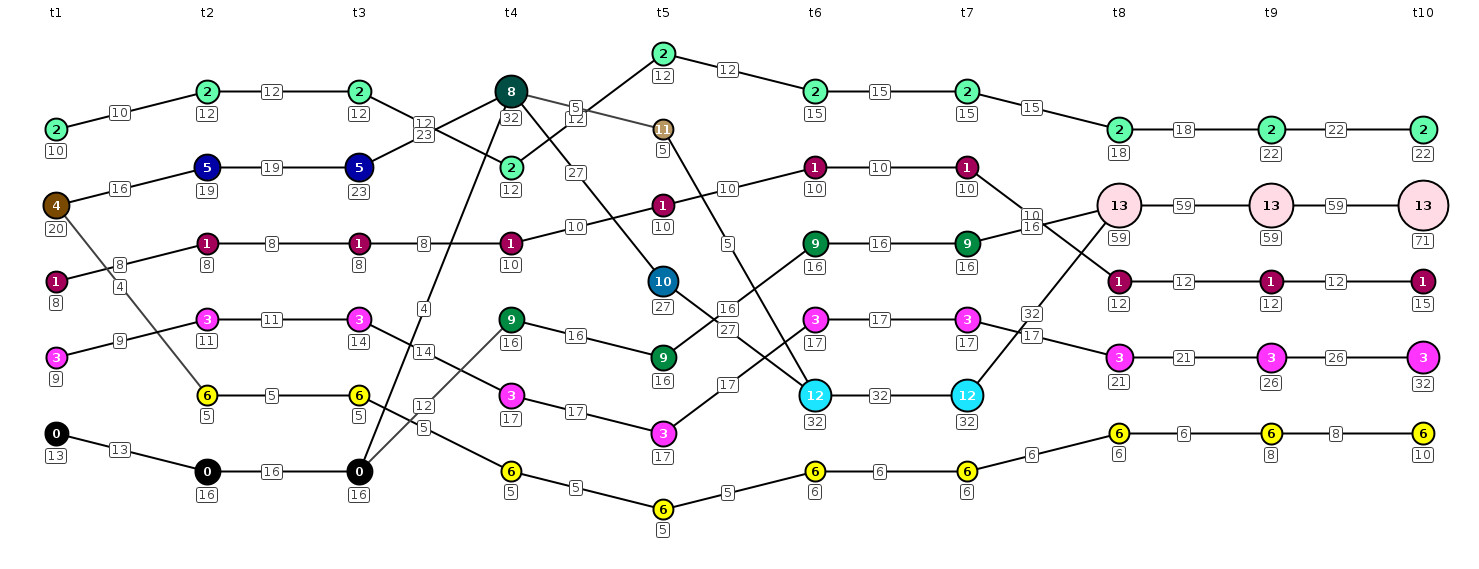#### Example of generated dynamic graph

A dynamic network spanning 10 timestamps starting with 5 communities. Vertex color corresponds to community membership. The layout is based on Kamada-Kawaï algorithm.

#### User interface of the software

The parameters are on the left panel. Graph visualisation and options in the center panel and the measures are displayed at the bottom. The community dynamics and degree distributions panels are availlable through tabs.

#### Dynamic of the communities

Evolution of the communities in a generated dynamic network. Each node corresponds to a community at a given timestamp and edges indicate that two consecutive communities share common vertices.

## Communities

• K
• Number of communities in the first graph
• n
• Number of vertices in the first graph
• Nb. Rep.
• Number of representents in each community. The higher is the value, the slower is the computation
• Theta
• Percentage of vertices assigned to a random community. The higher is this value, the less likely the community will be homogeneous w.r.t. the attributes

## Attributes

• Nb. Attr.
• Number of attributes associated to the vertices
• Dev. i
• Standard deviation of the ith attribute

## Edges

• Average degree
• Select if "Edges Within" or "Edges Between" parameters correspond to the average degree of the vertices of the maximum number of edges added to a newly inserted vertex
• Edges Within
• Maximum number of within community edges added to a newly inserted vertex
• Edges Between
• Maximum number of between community edges added to a newly inserted vertex
• MTE
• Minimum number of edges in the resulting graph (up to a graph where communities are cliques)

## Micro Dynamic

• Proba Micro
• The probability to perform a micro update operation
• The ratio of vertices created at each timestamp. When set to 1, the number of vertices is doubled at each timestamp
• Remove Vertex
• The ratio of vertices removed at each timestamp
• Update Attr.
• The ratio of vertices having their attribute values updated
• The ratio of edges inserted connecting two vertices in different communities
• Remove Btw. Edges
• The ratio of edges removed connecting two vertices in different communities
• The ratio of edges inserted connecting two vertices in the same communities
• Remove Wth. Edges
• The ratio of edges removed connecting two vertices in the same communities

## Macro Dynamic

• Timestamps
• The number of timestamp (i.e., the number of single graph generated)
• Proba Merge
• The probability to perform a merge operation at a single timestamp (i.e., merging two communities into a single one)
• Proba Split
• The probability to perform a split operation at a single timestamp (i.e., split one community into two)
• Proba Migrate
• The probability to perform a migrate operation at a single timestamp (i.e., migrate vertices from a community to either a new or an existing community)

## Attribute measures

• Observed homophily
• Ratio of edges connecting similar vertices w.r.t. their attribute values
• Expected homophily
• Ratio of pair of similar vertices among all possible pairs of vertices
• Within inertia
• Measure of the dispersion of the attribute values inside the communities. A low within inertia indicates that the communities are highly homogeneous w.r.t. the attribute values

## Structural measures

• Modularity
• The partition modularity measure has defined by M.E.J. Newman
• Average clustering coefficient
• Average clustering coefficient in the graph
• Random clustering coefficient
• Clustering coefficient in a Erdös-Renyi random graph having the same number of vertices and edges
• Average degree
• Average number of neighbours of the vertices
• Average shortest path length
• The average minimum number of hops required to reach two arbitrary vertices. It is not computed when the graph is formed by several disconnected components
• Diameter
• Length of the longest shortest path between any pair of vertices
• Nb. edges between
• Number of edges connecting two vertices belonging to different communities
• Nb. edges within
• Number of edges connecting two vertices belonging to the same community
• Nb. edges
• Total number of edges in the graph
• Graph output
• The generated dynamic network is output as a collection of files, one for each timestamp. The file extension is .graph and is splitted in three sections: the parameters, the vertices and the edges.
• Parameters
• This section starts with the line `# Parameters`. The parameters are output with a line starting with `#` followed by the parameter name and its value.
• Vertices
• This section starts with the line `# Vertices`. Each consecutive lines describes a vertex. A line consists of an integer corresponding to the vertex id, the list of its attribute values separated by a `;` and an integer corresponding to the vertex community id. Each value is separated by a `;`.

Example The line `1;0.31;0.49;2` corresponds to the vertex with id 1 associated to two attributes having values 0.31 and 0.49 and being in community 2.

• Edges
• This section starts with the line `# Edges`. Each consecutive lines corresponds to an edge. A line consists of two vertex ids separated by a `;`.

Example The line `1;4` corresponds to an edge between the vertex with id 1 and the vertex with id 4.

• Christine Largeron
• University of Saint-Étienne, France
• Pierre-Nicolas Mougel
• University of Saint-Étienne, France
• Oualid Benyahia
• University of Saint-Étienne, France
• Osmar Zaïane